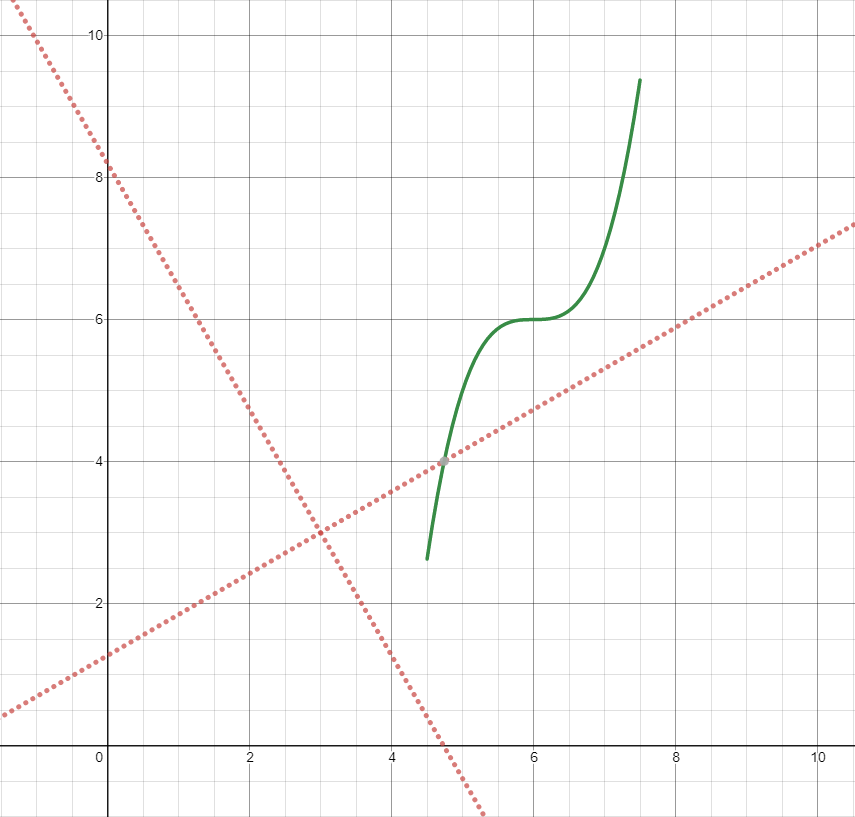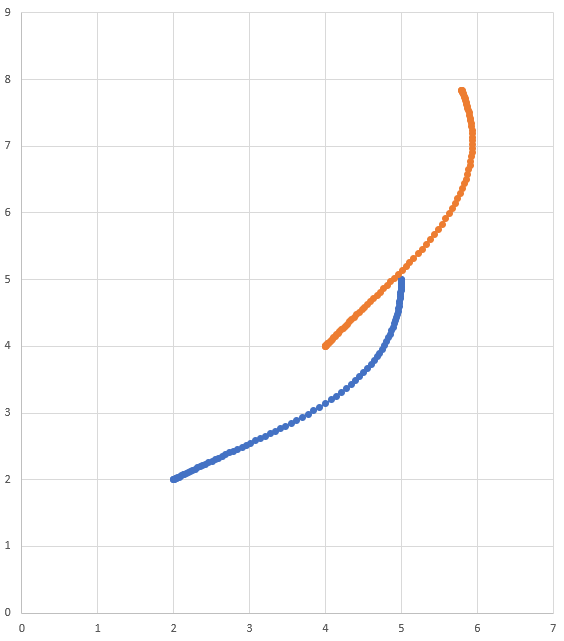Transforming Trajectories

Trajectories can be transformed from one coordinate system to another and moved within a coordinate system using the relativeTo and the transformBy methods. These methods are useful for moving trajectories within space, or redefining an already existing trajectory in another frame of reference.

Note

Neither of these methods changes the shape of the original trajectory.

The relativeTo Method

The relativeTo method is used to redefine an already existing trajectory in another frame of reference. This method takes one argument: a pose, (via a Pose2d object) that is defined with respect to the current coordinate system, that represents the origin of the new coordinate system.

For example, a trajectory defined in coordinate system A can be redefined in coordinate system B, whose origin is at (3, 3, 30 degrees) in coordinate system A, using the relativeTo method.

Pose2d bOrigin = new Pose2d(3, 3, Rotation2d.fromDegrees(30));
Trajectory bTrajectory = aTrajectory.relativeTo(bOrigin);In the diagram above, the original trajectory (aTrajectory in the code above) has been defined in coordinate system A, represented by the black axes. The red axes, located at (3, 3) and 30° with respect to the original coordinate system, represent coordinate system B. Calling relativeTo on aTrajectory will redefine all poses in the trajectory to be relative to coordinate system B (red axes).

The transformBy Method

The transformBy method can be used to move (i.e. translate and rotate) a trajectory within a coordinate system. This method takes one argument: a transform (via a Transform2d object) that maps the current initial position of the trajectory to a desired initial position of the same trajectory.

For example, one may want to transform a trajectory that begins at (2, 2, 30 degrees) to make it begin at (4, 4, 50 degrees) using the transformBy method.

Transform2d transform = new Pose2d(4, 4, Rotation2d.fromDegrees(50)).minus(trajectory.getInitialPose());
Trajectory newTrajectory = trajectory.transformBy(transform);In the diagram above, the original trajectory, which starts at (2, 2) and at 30° is visible in blue. After applying the transform above, the resultant trajectory’s starting location is changed to (4, 4) at 50°. The resultant trajectory is visible in orange.